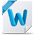# SPSS Statistics

View Only

## Change size of printed R ggplot in SPSS output

• #### 1.  Change size of printed R ggplot in SPSS output

Posted Tue September 26, 2023 04:52 PM

Hi there!  I am trying to use 'BEGIN PROGRAM R / END PROGRAM' to run a ggplot within SPSS (specifically, a clustered bar plot).  The syntax seems to be working well overall - except that I want the legend to be to the right of the plot while still being able to read the X-axis category labels, but right now it seems to be one or the other. I tried playing around with "theme(legend.position)" in the code below, as well as several other options that I thought might change the overall size of the plot so that the legend could simply be added to the right without having to "shrink" the space for the bars, but nothing has worked so far.

I am attaching 3 graphs (fake data) to illustrate what the ggplot looks like when the following "base" code is used and just the "theme(legend.position") input is varied:

BEGIN PROGRAM R.

#load spss data set as an R data frame called 'data'
data <- spssdata.GetDataFromSPSS()

#ggplot for clustered bar graph
pl <-  ggplot(data, aes(x=fct_inorder(Var1), y=Estimate, fill=fct_inorder(Var3))) +
theme(legend.position=c(0.75,0.92)) +
geom_bar(position="dodge", stat="identity", width=0.5) +
labs(x=data\$Label_Var1, fill=data\$Label_Var2, title="title")

#print the ggplot in SPSS output file
print(pl)

END PROGRAM.

Please note, I have also tried the following options to see if I could edit the overall size of the outputted ggplot, but they either produced an error (didn't run any plots) or produced the exact same plot as the "base" code (nothing changed):

·        ```{r fig.height = 10, fig.width = 5}<o:p></o:p>

·        options(repr.plot.width = 100, repr.plot.height = 5)<o:p></o:p>

·        png(filename = paste0("plot",".png",sep=""), width=10, height=5, unit="in", res=300)<o:p></o:p>

·        ggsave("testplot.png", width = 4, height = 3, dpi = 300)<o:p></o:p>

·        dev.new(width=10, height=5)<o:p></o:p>

·        print(pl, vp=grid::viewport(width=unit(10, 'inch'), height=unit(8, 'inch')))<o:p></o:p>